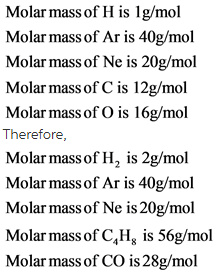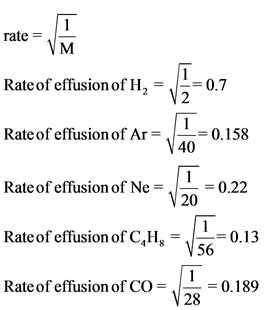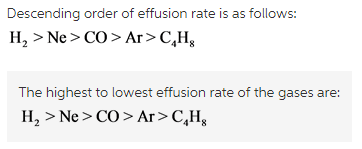# Rank from the highest to lowest effusion rate. To rank items as equivalent, overlap them

Rank the following gases in order of decreasing rate of effusion.
Rank from the highest to lowest effusion rate. To rank items as equivalent, overlap them.

• H2
• Ar
• Ne
• C4H8
• CO

Concepts and reason
Graham’s law of effusion can also be termed as Graham’s law of diffusion relates rate of effusion of gas and mass of the particle.

Fundamentals
Graham’s law of effusion states that, the rate of effusion of gas is inversely proportional to the square root of the mass of its particles.

Ratio of rate of effusion of two gases is written as follows:Explanation:
Molar mass of each gas is determined. Molar mass is defined as mass of the chemical element or a compound. Molar mass of a compound is calculated by adding the individual molar mass of the elements.Explanation:
The rate of effusion of each gases is found by substituting the molar mass of the respective gases in the rate effusion equation.Explanation:
The rate of effusion of the given gases is arranged from highest to lowest effusion rate. Hydrogen molecule has the highest effusion rate and hydrocarbon has the lowest effusion rate.